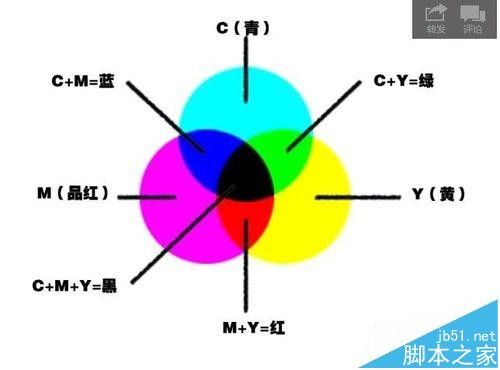# RGB与CMYK有什么区别？ RGB和CMYK颜色混合原理

RGB和CMYK颜色混合原理，RGB模式(颜色值介于0---255之间,三种颜色叠加可形成1670万种颜色)，RGB是一种色光混合模式R红色(R=255、G=0、B=0)

G绿色(R=0、G=255、B=0)

B蓝色(R=0、G=0、B=255)

RGB三种颜色相加(颜色混合模式为滤色)后为白色(R=255、G=255、B=255)

RGB三种颜色值都为0时为纯黑色.R(红色)+G(绿色)=黄色(R=255、G=255、B=0）

R(红色)+B(蓝色)=洋红或品红色(R=255、G=0、B=255)

G(绿色)+B(蓝色)=青色(R=0、G=255、B=255)CMYK模式(颜色值介于0-----100之间)

CMYK是一种颜料混合模式、也称减色模式,比较适合于打印.C青色(C=100、M=0、Y=0、K=0)

M洋红或品红(C=0、M=100、Y=0、K=0)

Y黄色(C=0、M=0、Y=100、K=0)

K黑色(C=0、M=0、Y=0、K=100)CMY三种颜色相加(颜色混合模式为正片叠底)后为黑色(C=100、M=100、Y=100)，这种黑色并不是100%的纯黑色。K黑色其实也并非100%的纯黑色，只不过是接近于黑色的褐色而已。K黑色与CMY或其它任何一种颜色相混合后都将变成由褐色到黑色的过渡色，即该值介于褐色和黑色之间。当CMYK颜色值都为0时为白色。

C+M=蓝色(C=100、M=100、Y=0)

C+Y=绿色(C=100、M=0、Y=100)

M+Y=红色(C=0、M=100、Y=100)